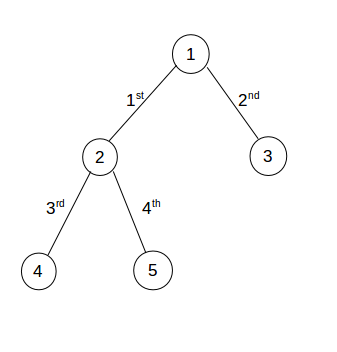Little Monk and Edge Count
Tag(s):

## Algorithms, Depth-first search, Easy-Medium, Graph, Graph Theory

Problem
Editorial
Analytics

Monk is struggling with a problem on graph and needs your help.

Given an undirected graph of N nodes and $N-1$ edges. There is a unique path from any node to any other node. For a given edge e, he needs to find number of pairs $(a,\;b)$, considering a and b as nodes of graph, such that e lies in the path between node a and node b.

Help monk for the same.

Input format:
First line of input contains two space separated integers, N $(1 \le N \le 10^5)$ and Q $(1 \le Q \le 10^5)$, denoting the number of nodes and number of queries.
Next $N-1$ lines contain two space separated integers, a and b, each, denoting there is an edge between a and b $(1 \le a, b \le N)$. $i^{th}$ line denotes $i^{th}$ edge.
Next Q lines contain one integer, x $(1 \le x \le N-1)$, denoting the $x^{th}$ edge.

Output format:
For each query, print the number of pairs of nodes $(a,\;b)$ such that $x^{th}$ edge lies in the path between a and b.

SAMPLE INPUT
5 4
1 2
1 3
2 4
2 5
1
2
3
4

SAMPLE OUTPUT
6
4
4
4

ExplanationFor $1^{st}$ query:
Number of pair such that $1^{st}$ edge lies in the path between them are $(1,\;2)$, $(1,\;4)$, $(1,\;5)$, $(3,\;2)$, $(3,\;4)$ and $(3,\;5)$.

For $2^{nd}$ query:
Number of pair such that $2^{nd}$ edge lies in the path between them are $(1,\;3)$, $(2,\;3)$, $(4,\;3)$, and $(5,\;3)$.

For $3^{rd}$ query:
Number of pair such that $3^{rd}$ edge lies in the path between them are $(1,\;4)$, $(2,\;4)$, $(3,\;4)$, and $(5,\;4)$.

For $4^{th}$ query:
Number of pair such that $4^{th}$ edge lies in the path between them are $(1,\;5)$, $(2,\;5)$, $(3,\;5)$, and $(4,\;5)$.

Time Limit: 1.0 sec(s) for each input file.
Memory Limit: 256 MB
Source Limit: 1024 KB
Marking Scheme: Marks are awarded when all the testcases pass.
Allowed Languages: Bash, C, C++, C++14, Clojure, C#, D, Erlang, F#, Go, Groovy, Haskell, Java, Java 8, JavaScript(Rhino), JavaScript(Node.js), Julia, Kotlin, Lisp, Lisp (SBCL), Lua, Objective-C, OCaml, Octave, Pascal, Perl, PHP, Python, Python 3, R(RScript), Racket, Ruby, Rust, Scala, Swift, Swift-4.1, TypeScript, Visual Basic

## CODE EDITOR

Initializing Code Editor...

## This Problem was Asked inChallenge Name

CodeMonk (Graph Theory Part I)

OTHER PROBLEMS OF THIS CHALLENGE
• Algorithms > Graphs
• Algorithms > Graphs
• Algorithms > Graphs
• Algorithms > Graphs